# An equilateral triangle inscribed in a rectangle

The following solution to the inscribed triangle puzzle is due to Peter Renz who communicated it to me on December 2016.

For the sake of completeness, let's begin with the statement of the puzzle. The figure below depicts a equilateral triangle $AEF$ inscribed in a rectangle in such a way that the two share a vertex. We wish to show that the area of the pink triangle ($ECF$) is the sum of the areas of the other two colored triangles.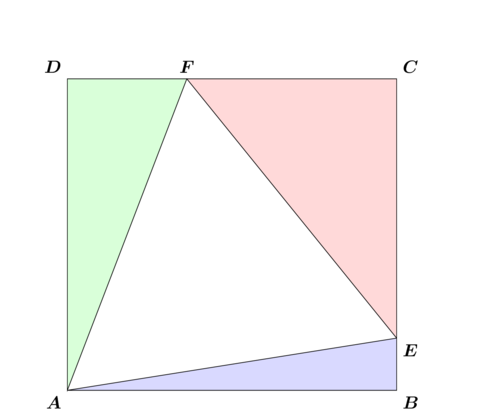As noted in the referring page (see the link above), a solution with the aid of trigonometry is quite straightforward. The purpose of this page is to present a solution in the style of Euclid, without appeal to trigonometry.

The following animation encapsulates Peter Renz's solution in its entirety.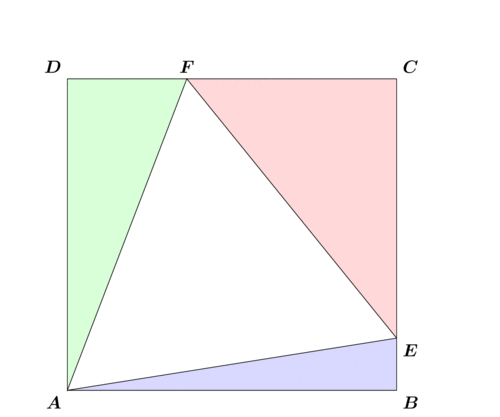The animation should be self-explanatory if you stare at it long enough. Nevertheless, I will now proceed to point out the reasoning through several still images extracted from that animation.
1. Construct a circle on the diameter $FE$, and then subdivide its boundary into six 60-degree wedges starting at the vertex $C$. Mark the division points $C$, $P$, $Q'$, $C'$, $P'$, and $Q$, as shown.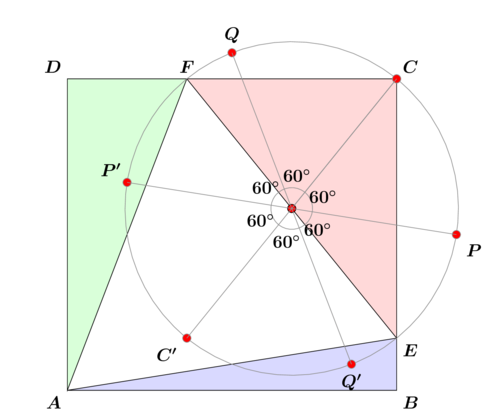2. Rotate the triangle $FDA$ about $F$ by 60 degrees to bring the edge $FA$ to coincide with $FE$.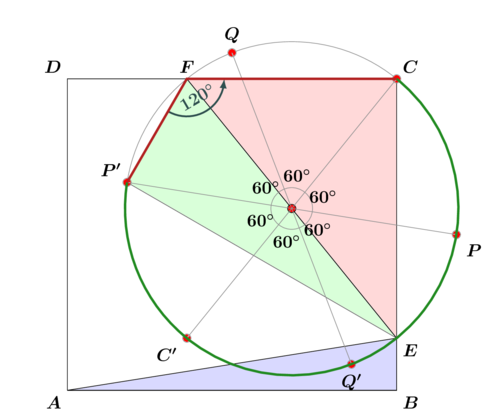The key observation is that the rotation moves the vertex $D$ into $P'$.
3. Further rotate the triangle about the circle's center by 180 degrees to place it in the $FPE$ position.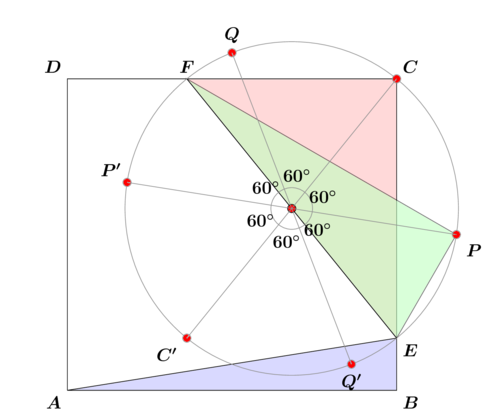4. Rotate the triangle $EBA$ about $E$ by 60 degrees to bring the edge $EA$ to coincide with $EF$. Then the vertex $B$ will move onto $Q'$ for reasons similar to those explained above.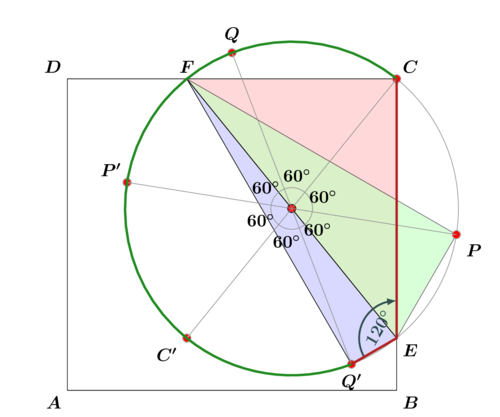5. The two red line segments in the figure below are parallel and of equal lengths by virtue of being the side and the “radius” of the regular hexagon (not shown) inscribed in the circle.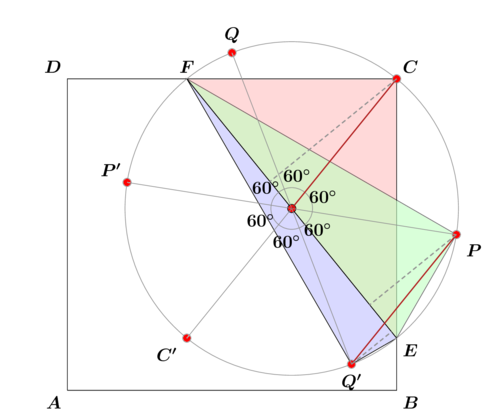The altitudes of the three triangles, dropped from the vertices $C$, $P$, and $Q'$ are shown in dashed lines. It should be clear that the altitude dropped from $C$ is equal in length to the sum of those of the other two altitudes. Since the three triangles share a common base, the area of one is the sum of the areas of the other two. Q.E.D.